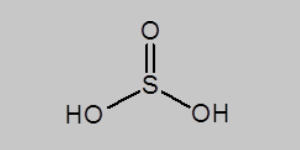# Sulfurous Acid Formula

The sulphurous or sulfurous acid formula should not be confused with the formula of sulphuric acid. While the formula may look similar but one of the main differences is that there is less number of oxygen atoms in sulfurous acid. Their physical and chemical properties also differ to some extent. Sulphurous acid is typically a dibasic or weak acid and is an aqueous solution of water and sulfur dioxide. It is colourless and has a pungent odour.

## Sulfurous Acid Chemical Formula

The formula of the sulphurous acid is given as H2SO3 where the molecule consists of two atoms of hydrogen, three atoms of oxygen and one atom of sulfur.

 Formula H2SO3 Molar Mass 82.07 g/mol Density 1.03 g/mL Boiling Point -60 °C

### Sulfurous Acid Structural Formula

Looking at the chemical structure of acid we can see that the molecule has the sulfur atom in the middle and three oxygen atoms are bonded where two oxygen atoms are formed by a single-bond and one has a double-bond. The two hydrogen atoms are connected by a single bond to the oxygen atoms. The sulfurous acid structural formula is normally written as;Keep visiting BYJU’S to know more about other chemical compound formulas as well as different chemistry concepts